# Statistics - Non-linear (effect|function|model)

Non-linear effect

The truth is almost never linear but often the linearity assumption is good enough. (Linearity is an approximation)

When its not (increasing in complexity):

offer a lot of flexibility, without losing the ease and interpretability of linear models.

## Product

In a linear model with two predictors, we will add another predictor: the interaction predictor which is:

• the product of the (two) predictors

We could then test for non-additive effects (ie to see if one predictor moderates the relationship between the other predictor and the outcome).

There might be an interaction or moderation effect between this predictors. If that is the case, then the interaction predictor would be a significant predictor of the outcome measure.

Discover More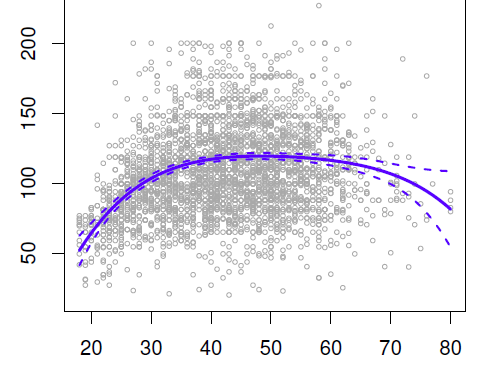Data Mining - (Global) Polynomial Regression (Degree)

polynomials regression Although polynomials are easy to think of, splines are much better behaved and more local. With polynomial regression, you create new variables that are just transformations...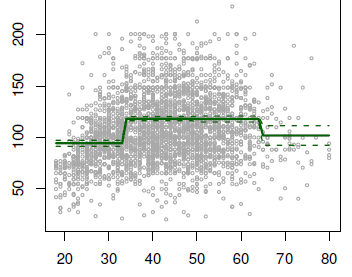Data Mining - Step Function (piecewise constants)

Step functions, are another way of fitting non-linearities. (especially popular in epidemiology and biostatistics) Continuous variable are cut into discrete sub-ranges and fit a constant model in...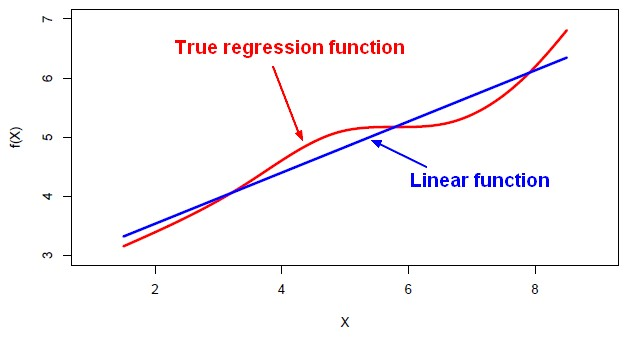Machine Learning - Linear (Regression|Model)

Linear regression is a regression method (ie mathematical technique for predicting numeric outcome) based on the resolution of linear equation. This is a classical statistical method dating back more...R - Multiple Linear Regression

Multiple linear regression with R functions such as lm Unstandardized Multiple Regression Regression analyses, standardized (in the z scale). The point is a short-cut to select all variables....R - Non-linear Effect Analysis

Non-linear Analysis with R. where: the quadratic is indicated by the power 2 (predictor1^2) As power has a meaning in this formula, the identity function (I) is used to protect it....Statistics - Generalized Linear Models (GLM) - Extensions of the Linear Model

The Generalized Linear Model is an extension of the linear model that allows for lots of different,non-linear models to be tested in the context of regression. GLM is the mathematical framework used in...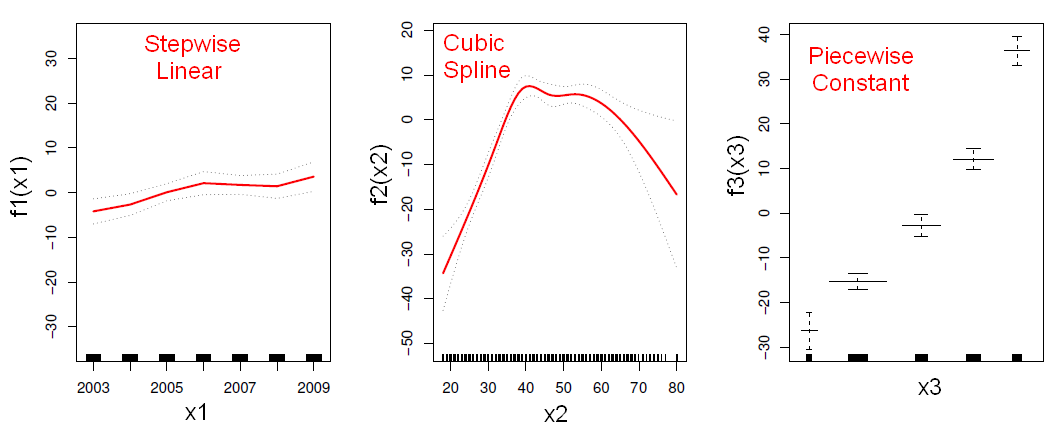Statistics - Generalized additive model (GAM)

In statistics, a generalized additive model (GAM) is a generalized linear model in which the linear predictor depends linearly on unknown smooth functions of some predictor variables, and interest focuses...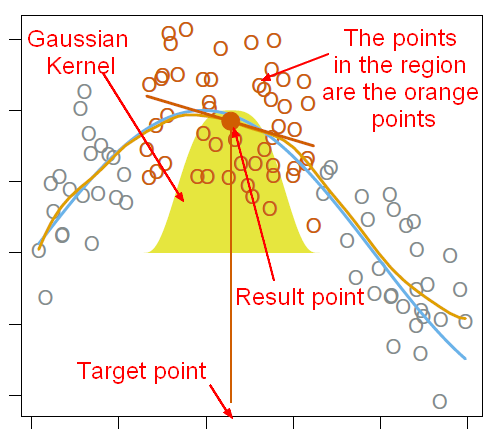Statistics - LOcal (Weighted) regrESSion (LOESS|LOWESS)

Popular family of methods called local regression that helps fitting non-linear functions just focusing locally on the data. LOESS and LOWESS (locally weighted scatterplot smoothing) are two strongly...